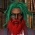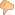Display replies flat, with oldest first Display replies flat, with newest first Display replies in threaded form Display replies in nested formainer Weissauer (University of Heidelberg) “Automorphic forms, L-functions and the Langlands conjectures”by Egor Pifagorov - Thursday, 17 May 2018, 01:39 AM СОВМЕСТНЫЙ КОЛЛОКВИУМ лаборатории им. Чебышева и ММИ им. Эйлера Четверг 24 мая ММИ им. Эйлера (Песочная наб., 10) Rainer Weissauer (University of Heidelberg) “Automorphic forms, L-functions and the Langlands conjectures” Despite the tremendous progress made during the 50 years since they were formulated, the Langlands conjectures remain mysterious until today. The conjectures, in their original form, relate finite dimensional representations $\rho$ of the absolute Galois group of $\mathbb Q$ to certain $L$-packets of automorphic representations $\pi$ of the adele groups $G({\mathbb A})$, for arbitrary reductive groups $G$ over $\mathbb Q$. This relationship should be, in a very precise way, controlled by a coincidence $L(\rho,s)=L(\pi,s)$ of $L$-series, and similarly of $\varepsilon$-factors, attached to $\rho$ respectively $\pi$. Furthermore, it is supposed that the correspondence respects the local-global principle. The analogous local Langlands conjecture, linking finite dimensional representations of the absolute Galois groups for completions $F$ of $\mathbb Q$ to smooth irreducible representations of $G(F)$, has been proved by Harris, Taylor and Henniart for the groups $G=Gl$ in 2001. However, beyond this not much is known for arbitrary $G$. We give a survey on this, including a discussion of some recent results on the Langlands correspondence in the case $G=GSp(4)$. Приглашаются все желающие!